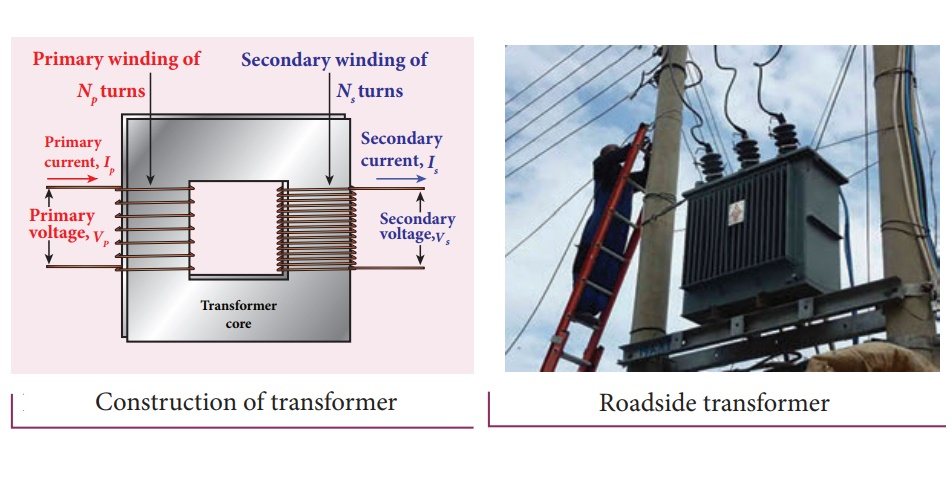Home | | Physics 12th Std | Construction and working Principle of transformer

# Construction and working Principle of transformerThe principle of transformer is the mutual induction between two coils. That is, when an electric current passing through a coil changes with time, an emf is induced in the neighbouring coil.

## TRANSFORMER

Transformer is a stationary device used to transform electrical power from one circuit to another without changing its frequency. The applied alternating voltage is either increased or decreased with corresponding decrease or increase of current in the circuit.

If the transformer converts an alternating current with low voltage into an alternating current with high voltage, it is called step-up transformer. On the contrary, if the transformer converts alternating current with high voltage into an alternating current with low voltage, then it is called step-down transformer.

Construction and working of transformer

## Principle

The principle of transformer is the mutual induction between two coils. That is, when an electric current passing through a coil changes with time, an emf is induced in the neighbouring coil.## Construction

In the simple construction of transformers, there are two coils of high mutual inductance wound over the same transformer core. The core is generally laminated and is made up of a good magnetic material like silicon steel. Coils are electrically insulated but magnetically linked via transformer core (Figure 4.37).

The coil across which alternating voltage is applied is called primary coil P and the coil from which output power is drawn out is called secondary coil S. The assembled core and coils are kept in a container which is filled with suitable medium for better insulation and cooling purpose.

## Working

If the primary coil is connected to a source of alternating voltage, an alternating magnetic flux is set up in the laminated core. If there is no magnetic flux leakage, then whole of magnetic flux linked with primary coil is also linked with secondary coil. This means that rate at which magnetic flux changes through each turn is same for both primary and secondary coils.

As a result of flux change, emf is induced in both primary and secondary coils. The emf induced in the primary coil εp is almost equal and opposite to the applied voltage υp and is given byThe frequency of alternating magnetic flux in the core is same as the frequency of the applied voltage. Therefore, induced emf in secondary will also have same frequency as that of applied voltage. The emf induced in the secondary coil εs is given bywhere N p and Ns are the number of turns in the primary and secondary coil respectively. If the secondary circuit is open, then εs = υs where υs is the voltage across secondary coil.This constant K is known as voltage transformation ratio. For an ideal transformer,

Input power υp ip = Output power υs is

where ip and is are the currents in the primary and secondary coil respectively.

Therefore,Equation 4.33 is written in terms of amplitude of corresponding quantities,i) If N s > N p ( or K >1), Vs >Vp  and I s < Ip .

This is the case of step-up transformer in which voltage is increased and the corresponding current is decreased.

ii) If N s < N p ( or K <1), Vs <Vp  and I s > Ip .

This is step-down transformer where voltage is decreased and the current is increased.

## Efficiency of a transformer:

The efficiency η of a transformer is defined as the ratio of the useful output power to the input power. ThusTransformers are highly efficient devices having their efficiency in the range of 96 – 99%. Various energy losses in a transformer will not allow them to be 100% efficient.

Study Material, Lecturing Notes, Assignment, Reference, Wiki description explanation, brief detail
12th Physics : Electromagnetic Induction and Alternating Current : Construction and working Principle of transformer |

Related Topics